﻿ On the Simpson’s Inequality for Convex Functions on the Co-Ordinates

### On the Simpson’s Inequality for Convex Functions on the Co-Ordinates

M. EMIN ÖZDEMIR, AHMET OCAK AKDEMIR, HAVVA KAVURMACIOPEN ACCESSPEER-REVIEWED

## On the Simpson’s Inequality for Convex Functions on the Co-Ordinates

M. EMIN ÖZDEMIR1, AHMET OCAK AKDEMIR2,, HAVVA KAVURMACI3

1Ataturk University, K.K. Education Faculty, Department of Mathematics, Erzurum, Turkey

2Ağri İbrahim Çeçen University, Faculty of Science and Letters, Department of Mathematics, AĞRI, Turkey

3Yüzüncü Yil University, Education Faculty, Department of Mathematics, Van, Turkey

### Abstract

In this paper, a new lemma is proved and inequalities of Simpson type are established for convex functions on the co-ordinates and bounded functions.

• ÖZDEMIR, M. EMIN, AHMET OCAK AKDEMIR, and HAVVA KAVURMACI. "On the Simpson’s Inequality for Convex Functions on the Co-Ordinates." Turkish Journal of Analysis and Number Theory 2.5 (2014): 165-169.
• ÖZDEMIR, M. E. , AKDEMIR, A. O. , & KAVURMACI, H. (2014). On the Simpson’s Inequality for Convex Functions on the Co-Ordinates. Turkish Journal of Analysis and Number Theory, 2(5), 165-169.
• ÖZDEMIR, M. EMIN, AHMET OCAK AKDEMIR, and HAVVA KAVURMACI. "On the Simpson’s Inequality for Convex Functions on the Co-Ordinates." Turkish Journal of Analysis and Number Theory 2, no. 5 (2014): 165-169.

 Import into BibTeX Import into EndNote Import into RefMan Import into RefWorks

### 1. Introduction

The following inequality is well-known in the literature as Simpson.s inequality:

Theorem 1. Letbe a four times continuously differentiable mapping on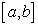and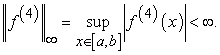Then the following inequality holds: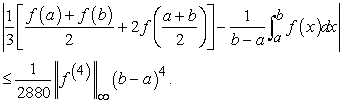For recent results on Simpson.s type inequalities see the papers [11-19].

Convexity on the co-ordinates can be given as following (see );

Let us consider the bidimensional intervalinwith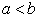andA functionwill be called convex on the co-ordinates if the partial mappings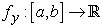and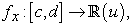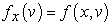are convex where defined for allandRecall that the mappingis convex on, if the following inequality;holds for all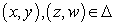and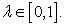In , Dragomir proved the following inequalities:

Theorem 2. Suppose thatis convex on the co-ordinates on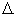Then one has the inequalities;(1.1)

The above inequalities are sharp.

Recently, several papers have been written on the convex functions on the co-ordinates. Similar results can be found in [1-9] and [20, 21, 22, 23].

In this paper, we will give Simpson-type inequalities for convex functions on the co-ordinates and bounded functions on the basis of the following lemma.

### 2. Main Results

To prove our main result, we need the following lemma.

Lemma 1. Letbe a partial differentiable mapping on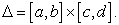If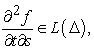then the following equality holds:(2.1)

whereand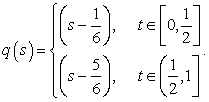Proof. Integrating by parts, we can writeBy integrating the right hand side of equality, we get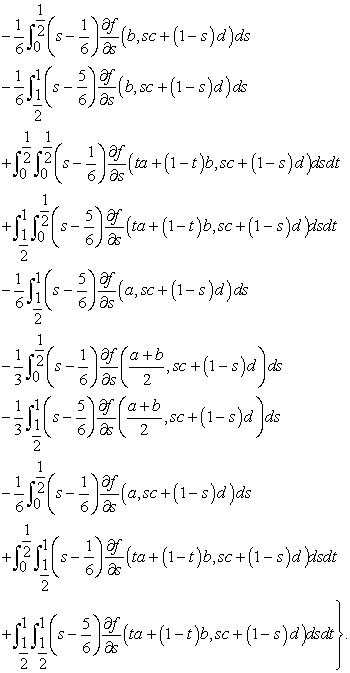Computing these integrals and using the change of the variable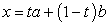and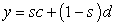for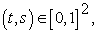then multiplying both sides with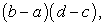we get the desired result.

Theorem 3. Letbe a partial differentiable mappingIfis a convex function on the co-ordinates onand,then the following inequality holds: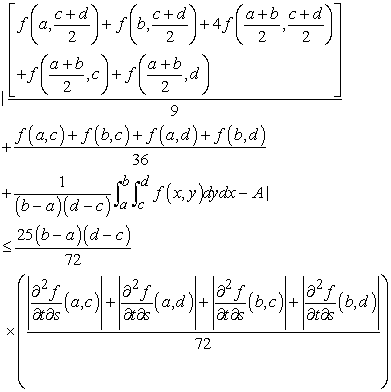whereProof. By using Lemma 1, we can writeSince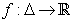is co-ordinated convex on,we get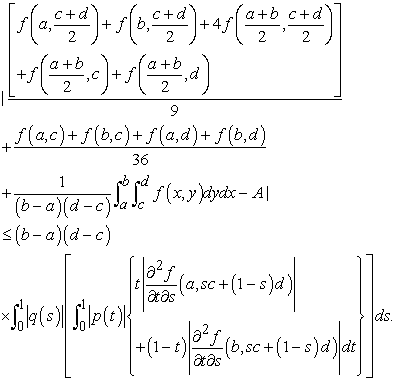Computing the integral in the right hand side of above inequality, we have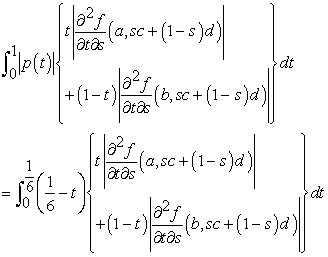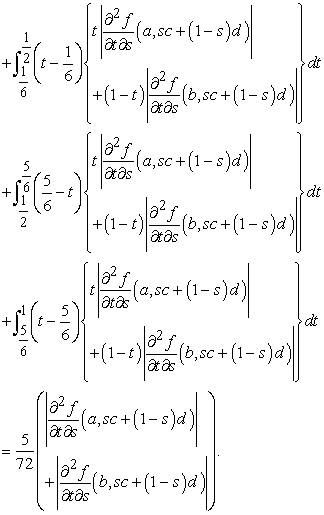We obtain(2.2)

By a similar argument for the above integral, we have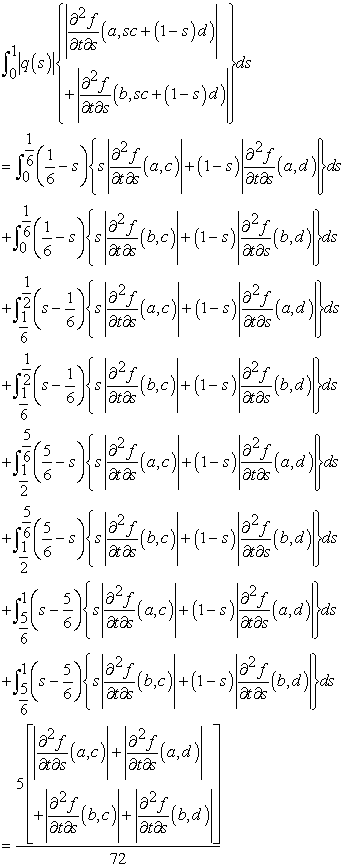(2.3)

If we use (2.3) in (2.2), we get the required result.

Theorem 4. Letbe a partial differentiable mapping onIfis bounded, i.e.,for allandThen the following inequality holds: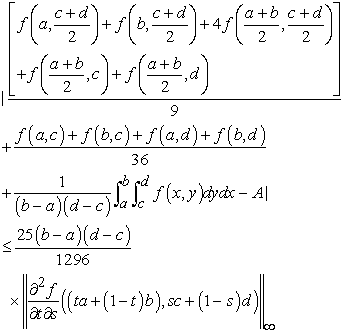where A is as in Theorem 3.

Proof. From Lemma 1 and using the property of modulus, we have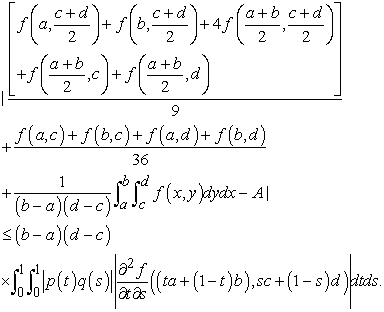Since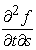is bounded, we have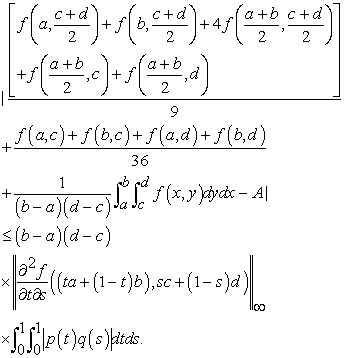(2.4)

By a simple calculation,(2.5)

If we use (2.5) in (2.4), we have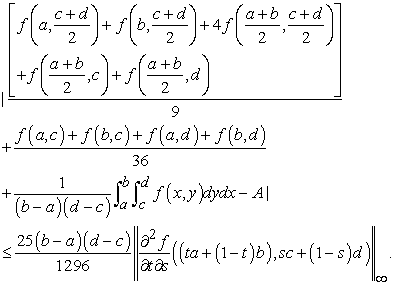This completes the proof.

### References

  Latif, M.A. and Alomari, M., On Hadamard-type inequalities for h-convex functions on the co-ordinates, International Journal of Math. Analysis, 3 (2009), no: 33, 1645-1656.In article  Latif, M.A. and Alomari, M., Hadamard-type inequalities for product two convex functions on the co-ordinates, International Mathematical Forum, 4 (2009), no: 47, 2327-2338.In article  Bakula, M.K. and Peµcari´c, J., On the Jensen.s inequality for convex functions on the co-ordinates in a rectangle from the plane, Taiwanese Journal of Math., 5 (2006), 1271-1292.In article  Alomari, M. and Darus, M., The Hadamard.s inequality for s-convex function of 2-variables on the co-ordinates, International Journal of Math. Analysis, 2 (2008) no: 13, 629-638.In article  Alomari, M. and Darus, M., Hadamard-type inequalities for s-convex functions, Interna-tional Mathematical Forum, 3 (2008), no: 40, 1965-1975.In article  Alomari, M. and Darus, M., Co-ordinated s-convex function in the first sense with some Hadamard-type inequalities, Int. Journal Contemp. Math. Sciences, 3 (2008), no: 32, 1557-1567.In article  Hwang, D.Y., Tseng, K.L. and Yang, G.S., Some Hadamard.s inequalities for co-ordinated convex functions in a rectangle from the plane, Taiwanese Journal of Mathematics, 11 (2007), 63-73.In article  Özdemir, M.E., Set, E. and Sarkaya, M.Z., Some new Hadamard.s type inequalities for co-ordinated m-convex and (α, m)-convex functions, Hacettepe J. of. Math. and St., 40, 219-229, (2011).In article  Sarkaya, M.Z., Set, E., Özdemir, M.E. and Dragomir, S. S., New some Hadamard’s type inequalities for co-ordinated convex functions, Tamsui Oxford Journal of Information and Mathematical Sciences, 28 (2), (2012), 137-152.In article  Dragomir, S.S., On the Hadamard’s inequality for convex functions on the co-ordinates in a rectangle from the plane, Taiwanese Journal of Mathematics, 5 (2001), no: 4, 775-788.In article  Sarkaya, M.Z., Set, E. and Özdemir, M.E., On new Inequalities of Simpson’s type for functions whose second derivatives absolute values are convex, RGMIA Res. Rep. Coll., 13 (1) (2010), Article 1.In article  Sarkaya, M.Z., Set, E. and Özdemir, M.E., On new inequalities of Simpson.s type for convex functions, RGMIA Res. Rep. Coll., 13 (2) (2010), Article 2.In article  Set, E., Özdemir, M.E. and Sarkaya, M.Z., On new inequalities of Simpson.s type for quasiconvex functions with applications, RGMIA Res. Rep. Coll., 13 (1) (2010), Article 6.In article  Sarkaya, M.Z., Set, E. and Özdemir, M.E., On new inequalities of Simpson.s type for s-convex functions, Computers & Mathematics with Applications, 60, 8 (2010).In article  Liu, B.Z., An inequality of Simpson type, Proc. R. Soc. A, 461 (2005), 2155-2158.In article CrossRef  Dragomir, S.S., Agarwal, R.P. and Cerone, P., On Simpson’s inequality and applications, J. of Ineq. and Appl., 5 (2000), 533-579.In article  Alomari, M., Darus, M. and Dragomir, S.S., New inequalities of Simpson.s type for s-convex functions with applications, RGMIA Res. Rep. Coll., 12 (4) (2009), Article 9.In article  Ujević, N., Double integral inequalities of Simpson type and applications, J. Appl. Math. and Computing, 14 (2004), no: 1-2, p. 213-223.In article  Zhongxue, L., On sharp inequalities of Simpson type and Ostrowski type in two independent variables, Comp. and Math. with Appl., 56 (2008), 2043-2047.In article CrossRef  Özdemir, M.E., Tunç, M. and Akdemir, A.O., On some new Hadamard-like inequalities for co-ordinated s-convex Functions, Facta Universitatis Series Mathematics and Informatics, Vol 28 No 3 (2013).In article  Özdemir, M.E., Akdemir, A.O. and Yldz, Ç., On co-ordinated quasi-convex functions, Czechoslovak Mathematical Journal, 62(137) (2012), 889-900.In article CrossRef  Özdemir, M.E., Kavurmac, H., Akdemir, A.O. and Avc, M., Inequalities for convex and s-convex functions on Δ = [a b]×[c,d], Journal of Inequalities and Applications, 2012, Published: 1 February 2012.In article  Özdemir, M.E., Yldz, Ç. and Akdemir, A.O., On some new Hadamard-type inequalities for co-ordinated quasi-convex functions, Hacettepe Journal of Mathematics and Statistics, 41(5) (2012), 697-707.In article  İşcan, İ., A new generalization of some integral inequalities for (α,m)-convex functions, Mathematical Sciences, 7(1) (2013), 1-8.In article CrossRef